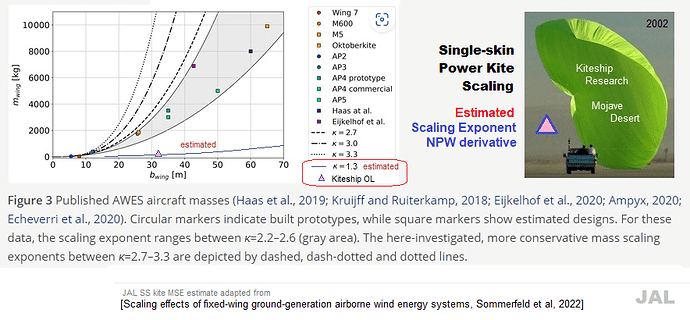# Scaling effects of fixed-wing ground-generation airborne wind energy systems

Markus Sommerfeld1, Martin Dörenkämper2, Jochem De Schutter3, and Curran Crawford1

• 1Institute for Integrated Energy Systems, University of Victoria, British Columbia, Canada
• 2Fraunhofer Institute for Wind Energy Systems (IWES), Oldenburg, Germany
• 3Systems Control and Optimization Laboratory IMTEK, University of Freiburg, Freiburg, Germany

Very thorough
Think I’ll stay working on rotary kite turbine designs for now though

2 Likes

A simple website to calculate the powers (exponents) of numbers (bases):

The paper is about rigid kites, so the more or less cube (cubed mass m³ following the volume) scaling law applies: (exponent) K = 2.7, or K = 3, or K = 3.3.
If the base = 10, we obtain about 501 with K = 2.7; then 1000 with K = 3; then about 1995 with K = 3.3. If the base = 2, we obtain respectively about 6.5; then 8; then about 9.85.

For flexible kites (by the numbers of the linked publication the mass increases a little more than the area, this increase slightly increasing as the wing becomes larger; and also take into account that the examples given in the publication may not be fully representative), an approximately square (squared mass m² following the area) scaling law applies, leading to K value of about 2.1-2.3 by my rough estimation, not as a result of the calculation of the numbers in the publication linked above.
If the base = 10, we obtain about 126 with K = 2.1, and about 199 with K = 2.3.
If the base is 2, we obtain respectively about 4.29 and about 4.92.

K value of 1.3 as stated on the comment above, would lead to a smaller increase in mass than the increase in area, which does not seem correct, compared to the quasi cubic K of 2.7, 3, and 3.3. Indeed if the base is 10, we obtain about only 19.95, and if the base = 2, we obtain about only 2.46, while with the exponent K = 2 we obtain respectively 100 and 4.

It is the reason why I consider an exponent slightly higher than 2 for flexible kites.

Note that there is a huge difference between K = 2.3 (the high end of my estimates by a ladle, for soft kites), and K = 2.7 (the low end of the estimates for a rigid kite).

2 Likes

If were using that chart to show wing scaling mass effects,
Let’s also use it to highlight the linear mass scaling associated with networking

for each demonstrated wing on the chart.
Using multiples of that wing type, increases the associated area and mass by that multiplier.
Little wings performance in network flocks scales better than the performance of single bigger kite wings.

1 Like

It does argue that the only way to matching > 20 MW single ground station installations is either multiple wings or soft wings.

1 Like

The significant increase of the exponent K when the kite area increases (for example the wing of 900 m² and 13.5 tons compared to the wing of 36 m² and 0.25 ton) could suggest that flexible kites also undergo scaling limits, but to a lesser degree than rigid wings.

For my part, I think that networks are a theoretical solution with a beginning of practice, but which lets foresee difficulties of management.

It remains to find ways to limit scalability problems, both for flexible wings (for example perhaps by using single skin kites) and rigid wings.

2 posts were merged into an existing topic: Slow Chat

My experience is that single line kites do generally scale and over a very wide range- provided their weight/area ratio stays relatively constant.

The smallest ram air flag kite is 0.75 m span, 0.35sq.m, 0.1kg. The largest is 45m span, 1250 sq.m, 360kg. The mini’s weight/area is around 0.28kg/sq.m, the mega’s is 0.29kg/sq.m. They both fly well, and very similarly, with good recovery and no weaving. The mega is more than 3,500 x’s the mini by area.

Single skin kites can also be scaled up until fabric and corded reinforcements reach their strength limit without any significant increases in weight/area- but in very large sizes would have far too much pull to be practical for kite event flying.

Another insight into how a single line flexible kite scales up. The weight/area is almost the same for small and giant kites, so that the exponent K = 2 by square law: the weight increases linearly with the area.

A further study on single skin power kites would be desirable.

2 Likes

I see you put the “corrected” graph (with K = 2) after my observations on the unlikely K = 1.3. History keeps both graphs I put below, after having renaming them by the history of the comment:For more clarity, it would have been necessary to mention this change in the body of the message.

That said I don’t think that K = 2 is a correct value. Peter Lynn’s giant kite is a static kite flying with very low wind speed. So K = 2 stands. But for power kites flying fast at high wind speeds, a slightly value is suitable, as I mentioned, and as it is confirmed by Storm Dunker page 532: https://www.researchgate.net/publication/290775361_Ram-air_Wing_Design_Considerations_for_Airborne_Wind_Energy : (0.25 ton, 1 ton, 2.25 tons, 4.5 tons, 13.5 tons, and finally 19 tons). The wing sizes were 36 m2, 102 m2, 250 m2, 350 m2, 900 m2, and 1,040 m2, respectively." K value increases when the wing area increases.